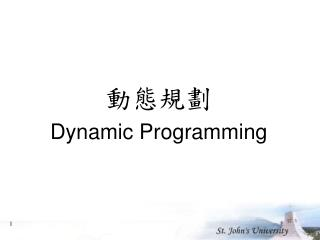Download Presentation# 動態規劃 Dynamic Programming - PowerPoint PPT PresentationI am the owner, or an agent authorized to act on behalf of the owner, of the copyrighted work described.
Download Presentation## 動態規劃 Dynamic Programming

Download Policy: Content on the Website is provided to you AS IS for your information and personal use and may not be sold / licensed / shared on other websites without getting consent from its author.While downloading, if for some reason you are not able to download a presentation, the publisher may have deleted the file from their server.

- - - - - - - - - - - - - - - - - - - - - - - - - - E N D - - - - - - - - - - - - - - - - - - - - - - - - - -
Presentation Transcript
1. 動態規劃 Dynamic Programming

2. 演算法方式總覽 • The Divide-and-Conquer Strategy (個各擊破) • binary Searching、Quick Sort…. • The Greedy Method(貪婪演算法) • Prim MST、Kruskal MST、Djikstra's algorithm • Dynamic Programming(動態演算法) • 二項是係數、矩陣連乘、最佳二元搜尋樹… • Trace Back(回溯) • 圖形著色、漢米爾迴路問題…. • Tree Searching Strategy(樹的追蹤)

3. 費氏數列(Fibonacci sequence) • Fibonacci sequence: 0 , 1 , 1 , 2 , 3 , 5 , 8 , 13 , 21 , … Fi = i if i 1 Fi = Fi-1 + Fi-2 if i 2 • Solved by a recursive program: • Much replicated computation is done. • It should be solved by a simple loop.

4. Dynamic Programming Dynamic Programming is an algorithm design method that can be used when the solution to a problem may be viewed as the result of a sequence of decisions 動態規劃是一種演算法的設計於當ㄧ個問題的解決方式是可以視為根據ㄧ連續的結果而得到。

5. 動態規劃介紹 • 當ㄧ個問題可以被分解成數各的性質相同的小問題。 • 會先計算較小的問題的結果，並且存儲。 • 如果有需要先前已經計算過的部份，就不需要重新計算，直接從先前存儲的結果中獲得。 • 由最小的問題開始計算，循序向上求取最後整個問提的答案。 • 是一種由下而上（bottom-up）的解決問題的方式。

6. 動態規劃設計步驟 • 建立一個遞迴機制，用它來求取ㄧ個問題經過切割後，所產生較小但性質相同的問題解。 • 用Bottom-up的方式解題，首先由最小的問題開始，逐步向上求取最後整各問題的解。

7. 動態規劃 VS個各擊破 • 相同 • 將ㄧ個問題切成數個較小問題來解。 • 相異 • 會先計算較小的問題並儲存計算結果(動態規劃) • 有計算過的小問題就無須重複計算(動態規劃) • Bottom-up的方式(動態規劃) • 盲目的遞迴計算(個各擊破)

8. 最短路徑(The shortest path) • To find a shortest path in a multi-stage graph • Apply the greedy method : the shortest path from S to T : 1 + 2 + 5 = 8

9. The shortest path in multistage graphs • e.g. • The greedy method can not be applied to this case: (S, A, D, T) 1+4+18 = 23. • The real shortest path is: (S, C, F, T) 5+2+2 = 9.

10. 動態規劃 • Dynamic programming approach (forward approach): • d(S, T) = min{1+d(A, T), 2+d(B, T), 5+d(C, T)} • d(A,T) = min{4+d(D,T), 11+d(E,T)} = min{4+18, 11+13} = 22.

11. d(B, T) = min{9+d(D, T), 5+d(E, T), 16+d(F, T)} = min{9+18, 5+13, 16+2} = 18. • d(C, T) = min{ 2+d(F, T) } = 2+2 = 4 • d(S, T) = min{1+d(A, T), 2+d(B, T), 5+d(C, T)} = min{1+22, 2+18, 5+4} = 9.

12. Backward approach • d(S, A) = 1 d(S, B) = 2 d(S, C) = 5 • d(S,D)=min{d(S,A)+d(A,D), d(S,B)+d(B,D)} = min{ 1+4, 2+9 } = 5 d(S,E)=min{d(S,A)+d(A,E), d(S,B)+d(B,E)} = min{ 1+11, 2+5 } = 7 d(S,F)=min{d(S,B)+d(B,F), d(S,C)+d(C,F)} = min{ 2+16, 5+2 } = 7

13. d(S,T) = min{d(S, D)+d(D, T), d(S,E)+ d(E,T), d(S, F)+d(F, T)} = min{ 5+18, 7+13, 7+2 } = 9

14. 練習 Find out the shortest path in the following graph.

15. 0-1 背包問題 假設有 n 個物品，令： S = {item1，item2，...，itemn} wi = itemi的重量 pi = itemi的價值 W = 背包的最大載重 其中，wi、Pi、W均為正整數，找出子集合 A 使得：

16. 貪婪解法範例 (1) 先拿價值最高的。 (2) 先拿重量最輕的。 (3) 先拿「價值/重量」比率最高的。 浪費5磅空間 最 大 載 重 30磅 20磅 \$140 20磅 20磅 \$60 \$50 10磅 10磅 5磅 5磅 拿取順序：1，3，2。 背包 貪婪 解法 最佳解 物品1 物品2 物品3

17. Example • n objects , weight：W1, W2, ,Wn profit：P1, P2, ,Pn capacity：M maximize： subject to：M xi = 0 or 1, 1in • e. g.

18. The multistage graph solution • The 0/1 knapsack problem can be described by a multistage graph.

19. 動態規劃 • The longest path represents the optimal solution: • x1=0, x2=1, x3=1 • = 20+30 = 50

20. 練習 0-1背包問題：求出下列最佳解

21. 資源分配問題 • The resource allocation problem • m resources, n projects profit Pi, j : j resources are allocated to project i. maximize the total profit.

22. The multistage graph solution • The resource allocation problem can be described as a multistage graph. • (i, j) : i resources allocated to projects 1, 2, …, j e.g. node H=(3, 2) : 3 resources allocated to projects 1, 2.

23. Find the longest path from S to T : (S, C, H, L, T), 8+5+0+0=13 2 resources allocated to project 1. 1 resource allocated to project 2. 0 resource allocated to projects 3, 4.

24. 練習 找出下列最佳的分配

25. 準則 • In summary, if a problem can be described by a multistage graph, then it can be solved by dynamic programming. • 如果一個問題可以轉成多階圖形問題，那ㄧ定可以用動態規劃解決

26. 二項式係數 分解

27. 遞迴推疊圖(The recursive stack) 呼叫過程: C4,2 B4,2 B3,1 B3,2 B2,1 B2,0 B2,1 B2,2 B2,0 B1,1 B2,0 B1,1

28. 設計動態規劃

29. 矩陣 B

30. 二項式係數(dynamic programming版)

31. 二項式係數時間複雜度

32. 練習 • 計算出 （6,2）之矩陣 B的值。

33. 動態規劃與個各擊破

34. 動態規劃和最佳化問題 定義： 最佳化原則(principle of optimality)要可以應用在一個問題上，他必須符合一個原則： 當一個問題存在著最佳解，則表示其所有的子問題也必存在著最佳解。

35. 連鎖矩陣相乘 Ex: 2x3矩陣乘 3x4矩陣

36. 連鎖矩陣相乘最佳化問題

37. 問題分析 • 使用暴力法(brute-force) • 找出具有最少乘法的組合 • 時間複雜度：指數(exponential)

38. 問題分析 • 矩陣相乘問題符合最佳化問題。 • 最佳化原則：如果有存在最佳的相乘順序，則此最佳相乘順序的任一子集合，也是最佳的相乘順序。 • 假設下列為六個矩陣的最佳相乘順序 • 則子集合亦是最佳相乘順序 • 使用動態規劃找尋最佳化的組合

39. 動態規劃

40. 陣列M規劃

41. Example

42. 最少乘法次數

43. 最少乘法次數(M 陣列)

44. 最少乘法次數(M 陣列)

45. 最少乘法次數(M 陣列)

46. 最少乘法次數演算法

47. 最少乘法次數的時間複雜度